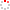NSSC OpenIR  > 空间科学部
 Spatial distribution of Kelvin-Helmholtz instability at low-latitude boundary layer under different solar wind speed conditions Li, W. Y.; Guo, X. C.; Wang, C.; Li, WY (reprint author), Chinese Acad Sci, State Key Lab Space Weather, Ctr Space Sci & Appl Res, Beijing 100190, Peoples R China. Department 空间科学部 Source Publication JOURNAL OF GEOPHYSICAL RESEARCH-SPACE PHYSICS2012 Volume 117Pages:A08230 ISSN 0148-0227 Language 英语 Abstract Using the PPMLR-MHD global simulation model, we examined the Kelvin-Helmholtz (K-H) instability at the low-latitude boundary layer (LLBL) under northward interplanetary magnetic field (IMF) conditions with various solar wind speeds (400, 600, and 800 km/s). The spatial distribution of the K-H wave power in the equatorial plane shows two distinct power populations, referring to the two modes of K-H surface waves. The spatial evolution of K-H instability at the boundary layer is classified into four phases: quasi-stable, exponential growth, linear growth, and nonlinear phases. The boundary layer is quasi-stable near the subsolar point region. The K-H instability starts at about 30 degrees longitude, and grows exponentially with a spatial growth rate of 0.28 similar to 0.87 R-E(-1) until similar to 45 degrees longitude where the vortices fully develop. At larger longitudes, the instability grows linearly, while the vortices grow in size. From similar to 80 degrees longitude to the distant magnetotail, the K-H instability develops nonlinearly and the vortices roll up. The wave frequency, wavelength, and phase speed are given at various spatial points. Model results show that the higher solar wind speed generates K-H waves with higher frequency under the northward IMF, and the wavelengths and the phase speeds increase with the increase of the longitude. Moreover, we made a comparison of the K-H wave periods on Earth's, Mercury's and Saturn's magnetopauses by a proposed prediction method.; Using the PPMLR-MHD global simulation model, we examined the Kelvin-Helmholtz (K-H) instability at the low-latitude boundary layer (LLBL) under northward interplanetary magnetic field (IMF) conditions with various solar wind speeds (400, 600, and 800 km/s). The spatial distribution of the K-H wave power in the equatorial plane shows two distinct power populations, referring to the two modes of K-H surface waves. The spatial evolution of K-H instability at the boundary layer is classified into four phases: quasi-stable, exponential growth, linear growth, and nonlinear phases. The boundary layer is quasi-stable near the subsolar point region. The K-H instability starts at about 30 degrees longitude, and grows exponentially with a spatial growth rate of 0.28 similar to 0.87 R-E(-1) until similar to 45 degrees longitude where the vortices fully develop. At larger longitudes, the instability grows linearly, while the vortices grow in size. From similar to 80 degrees longitude to the distant magnetotail, the K-H instability develops nonlinearly and the vortices roll up. The wave frequency, wavelength, and phase speed are given at various spatial points. Model results show that the higher solar wind speed generates K-H waves with higher frequency under the northward IMF, and the wavelengths and the phase speeds increase with the increase of the longitude. Moreover, we made a comparison of the K-H wave periods on Earth's, Mercury's and Saturn's magnetopauses by a proposed prediction method. Indexed By SCI Funding Project 中国科学院空间科学与应用研究中心 Document Type 期刊论文 Identifier http://ir.nssc.ac.cn/handle/122/3220 Collection 空间科学部 Corresponding Author Li, WY (reprint author), Chinese Acad Sci, State Key Lab Space Weather, Ctr Space Sci & Appl Res, Beijing 100190, Peoples R China. Recommended CitationGB/T 7714 Li, W. Y.,Guo, X. C.,Wang, C.,et al. Spatial distribution of Kelvin-Helmholtz instability at low-latitude boundary layer under different solar wind speed conditions[J]. JOURNAL OF GEOPHYSICAL RESEARCH-SPACE PHYSICS,2012,117:A08230. APA Li, W. Y.,Guo, X. C.,Wang, C.,&Li, WY .(2012).Spatial distribution of Kelvin-Helmholtz instability at low-latitude boundary layer under different solar wind speed conditions.JOURNAL OF GEOPHYSICAL RESEARCH-SPACE PHYSICS,117,A08230. MLA Li, W. Y.,et al."Spatial distribution of Kelvin-Helmholtz instability at low-latitude boundary layer under different solar wind speed conditions".JOURNAL OF GEOPHYSICAL RESEARCH-SPACE PHYSICS 117(2012):A08230.
 Files in This Item: File Name/Size DocType Version Access License 2012117A08230.pdf（3373KB） 开放获取 CC BY-NC-SA Application Full Text
 Related Services Recommend this item Bookmark Usage statistics Export to Endnote Google Scholar Similar articles in Google Scholar [Li, W. Y.]'s Articles [Guo, X. C.]'s Articles [Wang, C.]'s Articles Baidu academic Similar articles in Baidu academic [Li, W. Y.]'s Articles [Guo, X. C.]'s Articles [Wang, C.]'s Articles Bing Scholar Similar articles in Bing Scholar [Li, W. Y.]'s Articles [Guo, X. C.]'s Articles [Wang, C.]'s Articles Terms of Use No data! Social Bookmark/Share